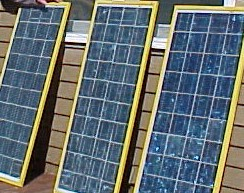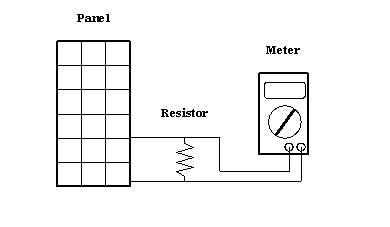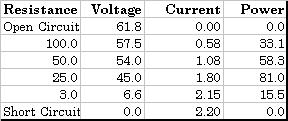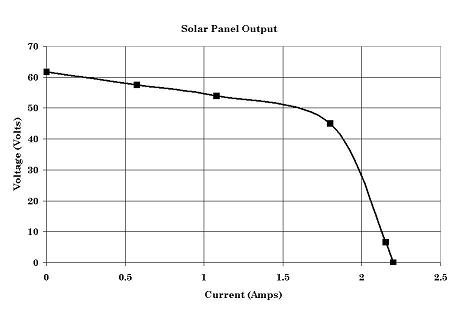MTM Scientific, Inc: How to Measure the Power Output of Solar PanelsA photovoltaic solar electric panel generates DC power when it is exposed to sunlight. A natural question to ask is 'How much power?' Here we explain how to test a solar panel for output and how to determine the maximum power point.

A solar panel is a generator of DC electrical power. DC electrical power wattage is the product of voltage and current.

DC Watts = Voltage X Current

Power is measured in units of Watts, (Symbol W)

Voltage is measured in units of Volts, (Symbol V)

Current is measured in units of Amps, (Symbol I)

The power generated by any solar panel in full sunlight depends on the resistance of the electrical load connected to it. Resistance is measured in units of Ohms. The relationship between current, voltage and resistance is referred to as Ohm's law. The current through a resistor is the applied voltage divided by the resistance.

Current = Voltage / Resistance

The method of measuring the power output of a solar panel is to connect resistors of various values to the panel and measure the voltage. The measurements can be used to calculate the power output. The same measurements can be used to plot the power output and create a performance graph for the panel.

A digital multimeter for measuring DC voltage is required for this test. An assortment of power resistors is also needed. In practice, each power resistor is connected to the panel and the voltage is measured with the meter, as shown in the drawing.The meter is also used to measure the open circuit voltage and the short circuit current. Here is a table of data from a set of measurement on 3 large solar panels connected in series. In this example power resistors of 100, 50, 25 and 3 ohms were used.  The current through each resistor is calculated by dividing the measured voltage by the resistance.  The power is calculated by multiplying the voltage times the current.Note that no power is generated when the open circuit voltage and short circuit current is measured.  A more intuitive view of the data can be gained by plotting a graph of the voltage versus current.The output of this solar panel shows a characteristic behavior that is common to all solar panels.  The maximum power is generated at the operating point which forms the 'knee' in the curve. In our case, this is at approximately 81 watts, corresponding to a load resistance of 25 ohms. The maximum power point is where the product of current and voltage is a maximum. Expressed graphically, the maximum power point it where the largest area rectangle can be formed beneath the curve.

Testing a solar panel requires an assortment of power resistors capable of handling the power. In most cases, ordinary 1/4 and 1/2 watt resistors are NOT suitable for this type of measurement. Small resistors overheat and burn out. Power resistors are made to handle more wattage, although they will become hot over time and can burn out too. Adjustable power resistors (called rheostats) are  also useful, although they require a meter for measuring the resistance after each adjustment. The simplest way to avoid problems with overheating is to use high wattage resistors and to make individual measurements as quickly as possible.

The power output of solar panels can be increased by tracking the position of the sun, as described in our Solar Tracker E-Booklet.

Electric DC gearhead motors can be used as a DC generator, and can be tested in a similar way, as described in our Motor Generator E-Booklet.